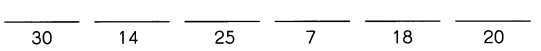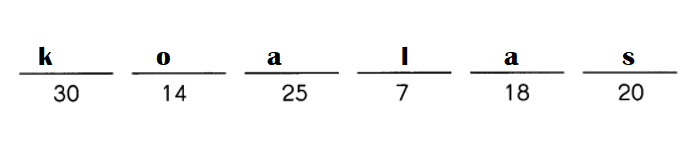# Math in Focus Grade 4 Chapter 6 Practice 7 Answer Key Fraction of a Set

Practice the problems of Math in Focus Grade 4 Workbook Answer Key Chapter 6 Practice 7 Fraction of a Set to score better marks in the exam.

## Math in Focus Grade 4 Chapter 6 Practice 7 Answer Key Fraction of a Set

Check (✓) the box next to the group of shapes that show $$\frac{3}{5}$$ shaded.

Question 1.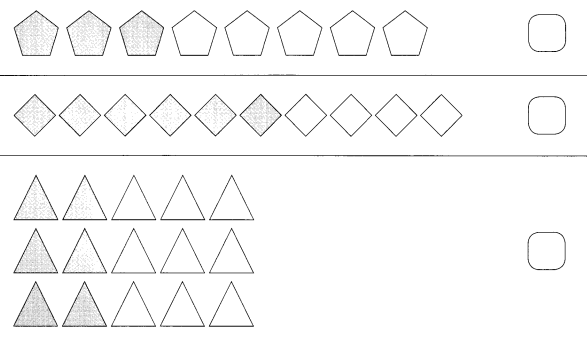Triangles and squares are shaded in $$\frac{3}{5}$$.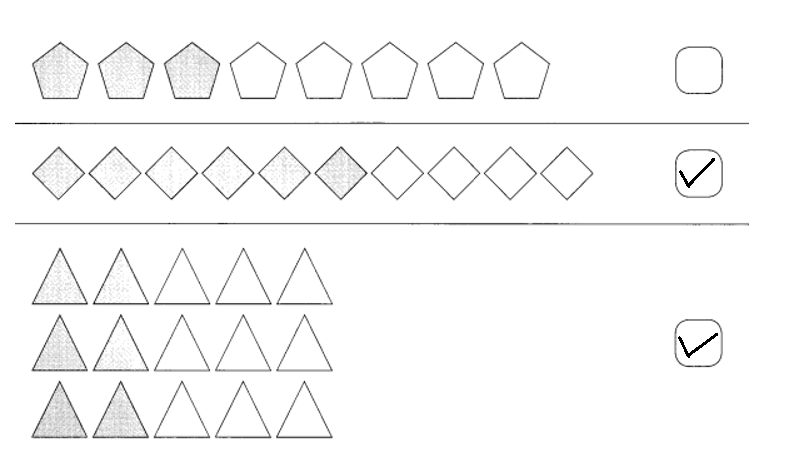Explanation:
Total number of rhombus = 8.
Number of rhombus shaded = Total number of rhombus × $$\frac{3}{5}$$
= 8 × $$\frac{3}{5}$$
= $$\frac{24}{5}$$
Total number of squares = 10.
Number of squares shaded = Total number of squares × $$\frac{3}{5}$$
= 10 × $$\frac{3}{5}$$
= 2 × 3
= 6.
Total number of triangles = 10.
Number of triangles shaded = Total number of triangles × $$\frac{3}{5}$$
= 15 × $$\frac{3}{5}$$
= 3 × 3
= 6.

Example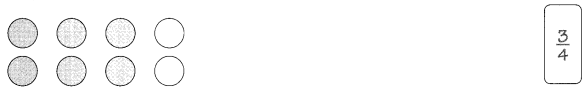Question 2.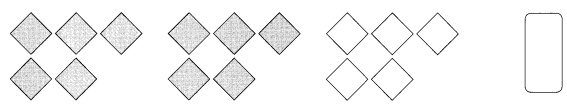Fraction of shaded squares = 2 ÷ 3 or $$\frac{2}{3}$$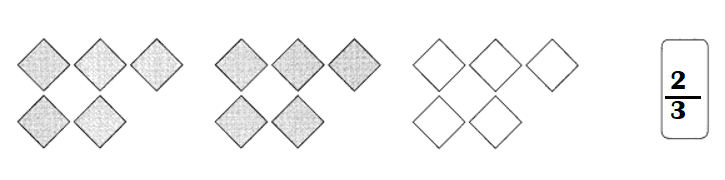Explanation:
Total number of squares = 15.
Number of squares shaded = 10.
Fraction of shaded squares = Number of squares shaded ÷ Total number of squares
= 10 ÷ 15
= 2 ÷ 3 or $$\frac{2}{3}$$

Question 3.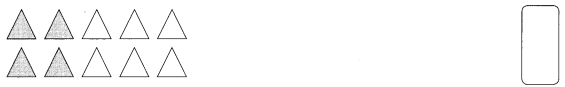Fraction of shaded triangles = 2 ÷ 5 or $$\frac{2}{5}$$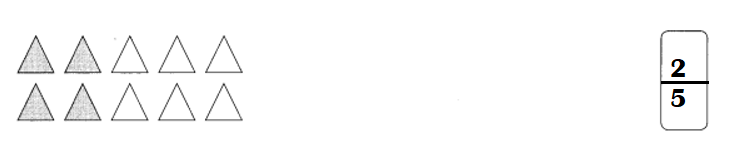Explanation:
Total number of triangles = 10.
Number of triangles shaded = 4.
Fraction of shaded triangles = Number of triangles shaded ÷ Total number of triangles
= 4 ÷ 10
= 2 ÷ 5 or $$\frac{2}{5}$$

Example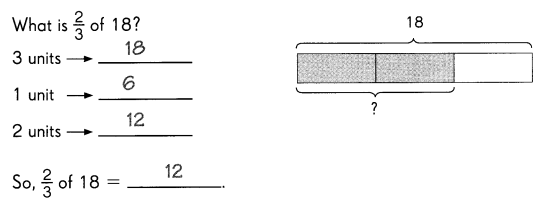Question 4.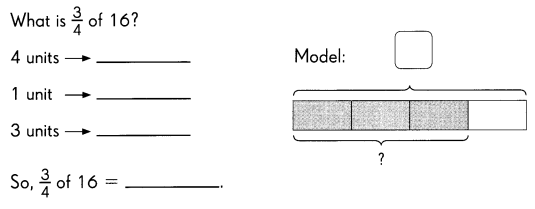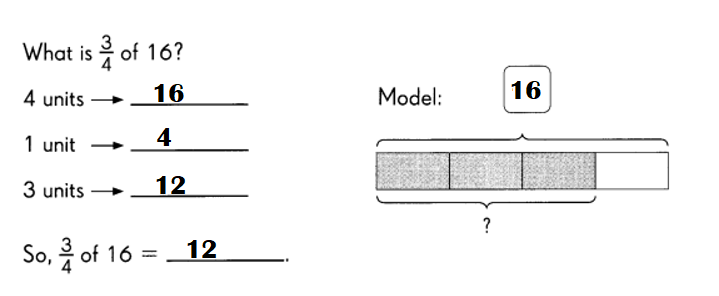Explanation:
3 ÷ 4 × 16 = 3 × 4 = 12.
4 units = 16.
1 unit = 4.
3 units = 12.
So, $$\frac{3}{4}$$× 16 = 3 × 4 = 12.

Question 5.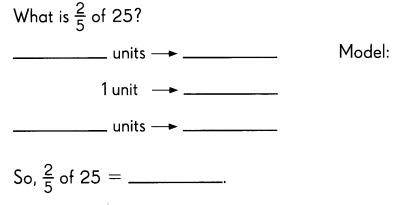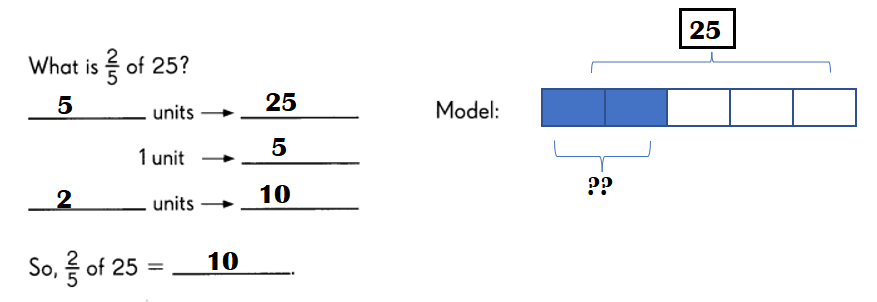Explanation:
5 units = 25.
1 unit = 5.
2 units = 10.
So, $$\frac{2}{5}$$ × 25 = 10.

Question 6.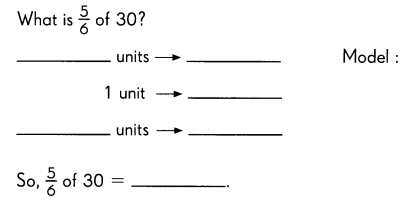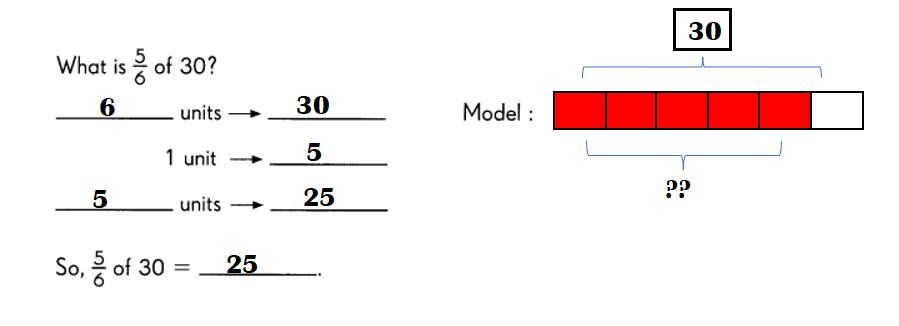Explanation:
6 units = 30.
1 unit = 5.
5 units = 25.
So, $$\frac{5}{6}$$× 30 = 25.

Solve.

Example
$$\frac{2}{3}$$ × 15
$$\frac{2}{3}$$ of 15 is 10

Question 7.
$$\frac{3}{4}$$ × 12
$$\frac{2}{3}$$ of 12 is ____
$$\frac{3}{4}$$ × 12
$$\frac{2}{3}$$ of 12 is 8.

Explanation:
$$\frac{3}{4}$$ × 12
$$\frac{2}{3}$$ of 12 = 2 × 4 = 8.

Question 8.
$$\frac{2}{5}$$ × 20
$$\frac{2}{5}$$ of 20 is ____
$$\frac{2}{5}$$ × 20
$$\frac{2}{5}$$ of 20 is 8.

Explanation:
$$\frac{2}{5}$$ × 20
$$\frac{2}{5}$$ of 20 = 2 × 4 = 8.

Question 9.
$$\frac{6}{7}$$ × 42
$$\frac{6}{7}$$ of 42 is ____
$$\frac{6}{7}$$ × 42
$$\frac{6}{7}$$ of 42 is 36.

Explanation:
$$\frac{6}{7}$$ × 42
$$\frac{6}{7}$$ of 42 = 6 × 6 = 36.

Fill in the blanks to solve each problem.

Example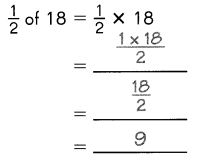Question 10.
$$\frac{2}{3}$$ of 24 = $$\frac{2}{3}$$ × ____
= ____
= ____
= ____
$$\frac{2}{3}$$ of 24 = $$\frac{2}{3}$$ × 24 = 16.

Explanation:
$$\frac{2}{3}$$ of 24 = $$\frac{2}{3}$$ × 24
= 2 × 8
= 16.

Question 11.
$$\frac{3}{4}$$ of 32 = ___ × 32
= ____
= ____
= ____
$$\frac{3}{4}$$ of 32 = $$\frac{3}{4}$$ × 32 = 24.

Explanation:
$$\frac{3}{4}$$ of 32 = $$\frac{3}{4}$$ × 32
= 3 × 8
= 24.

Write each answer in the box. Then solve the riddle.

Question 12.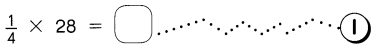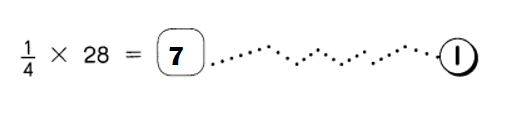Explanation:
$$\frac{1}{4}$$ × 28 = 1 × 7 = 7.

Question 13.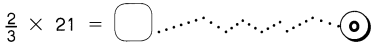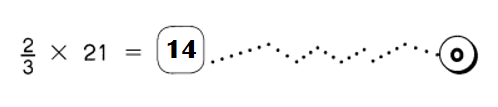Explanation:
$$\frac{2}{3}$$ × 21 = 2 × 7 = 14.

Question 14.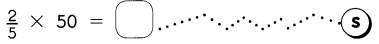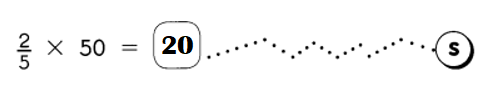Explanation:
$$\frac{2}{5}$$ × 50 = 2 × 10 = 20.

Question 15.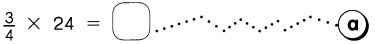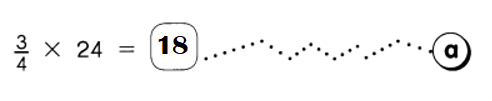Explanation:
$$\frac{3}{4}$$ × 24 = 3 × 6 = 18.

Question 16.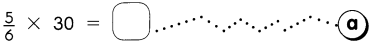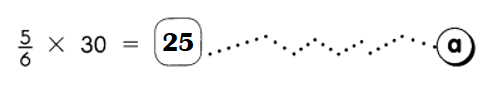Explanation:
$$\frac{5}{6}$$ × 30 = 5 × 5 = 25.

Question 17.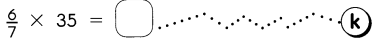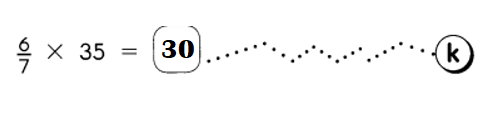Explanation:
$$\frac{6}{7}$$ × 35 = 6 × 5 = 30.

18. Which animals often sleep about 18 to 20 hours a day?
Write the letters that match the answers to find out.